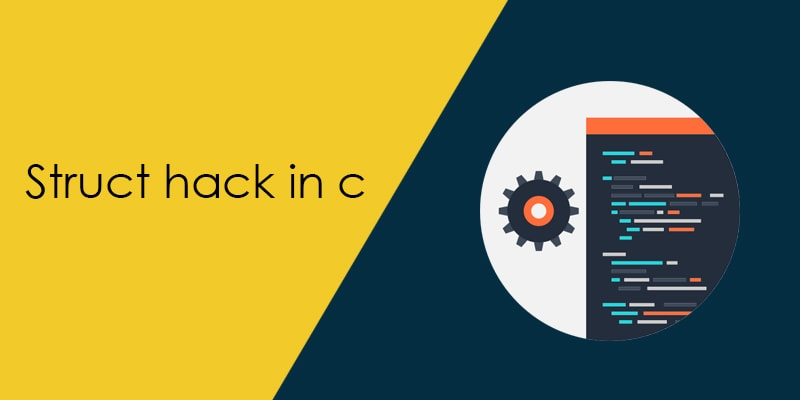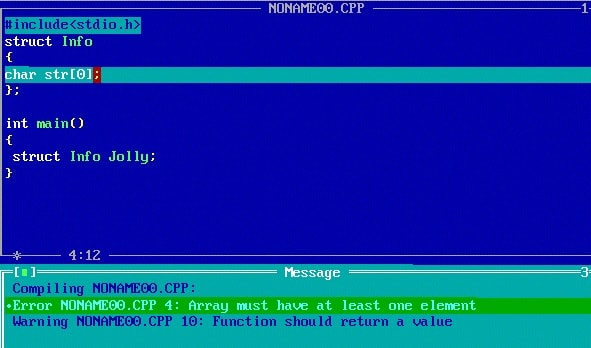# What is importance of struct hack in c?Previously when C99 had not introduced flexible array, people used the technique of struct hack to create the flexible length member. The struct hack technique gives the permission to the user to create a variable length member in the structure.

In struct hack techniques, we need to create an array whose length is 0 (some compiler does not support the 0 size array).When we create a zero size array then structure becomes the incomplete type. Basically, an incomplete type structure is a type that has lack of information about its member.

Let’s take an example to understand the techniques of struct hack,

As I have mentioned above, if we create an incomplete type member in the structure, the structure becomes incomplete types and this technique is called struct hack.

In below structure I am creating a character array to store student name, I am giving the length of the array 0 (some compiler does not support 0 length array, in that scenario we have to take the length of the array 1).

#### What is the size of the struct hack?

When we calculate the size of the structure then we found that compiler does not include the size of the 0 length array (Name). So if we assume the size of the integer is 4 bytes, the size of the structure become (Here we assume alignment is 4 bytes) 8 bytes.

sizeof(sStudentInfo) = sizeof(RollNumber)+ sizeof(TotalMarks) + sizeof(Name) ;
So output will be 4 + 4 + 0 = 8 bytes.

#### Sample program to calculate the size of the structure

In below example code I am calculating the size of the structure which contains 0 length array member.

Output:  Size of structure = 12

#### Why is struct hack required?

Let’s take an example to understand the above question. First I want to declare a structure which contains the information (price, name, expiry date ..etc) of medicine.

In above structure, the name of the medicine should be dynamic. If the name of the medicine is less then MaxSize, it causes the memory loss but if the medicine name greater then the MaxSize, you could be faced the code crashing issues. With the help of struct hack we can resolve this issues and able to create the variable length array whose size could be changed as per the requirements.

#### Why not use a pointer?

Using the pointer we can also create the dynamic length array but problem is that in pointers take extra (4 or 8 bytes depending on the system) memory. When we have created a pointer to the structure then we have to explicitly allocate the memory for the pointers but if we used struct hack then there is no need to allocate memory again for the array.

Let’s see an example to the better understanding.

Suppose there are two structure one contains ” char * ” and second contains the “0 length array”, both structure is used to store the name and number of characters within the name.

• In sNameInfo1 the character data could be anywhere in memory and pointed by the pcName but in sNameInfo2 character data is in inside the structure.
• If we create a pointer to sNameInfo1 you need to take care of allocating and freeing of character pointer in addition of struct itself but with pointer to sNameInfo2, we need allocating and freeing the struct itself because everything is packaged together.

example,

Many places where struct hack makes sense, I am explaining some scenario where struct hack is very useful.

• Suppose you are working on a file where you need to store the user information. If you are using struct hack, you need to call write function only once to write all the data in the file because structure and character payload packaged together.It is not possible if you create character pointer in place of the 0 length array.
• Suppose you want to send the data to the server (TCP/IP), the server expects the received message in the form of the data and length.Using the struct hack you can send the whole data in the single call of send (Name of send function could be anything depending on the system) library function.In place of the array if you used pointer then you will need to calls send function twice, otherwise, you will send the address of the pointer in place of the actual data.
• If you will use an array in place of the pointer, you can use memcpy to copy the data from the one object to another one without any culprit.1.Akshay Immanuel says:
1.Amlendra says: